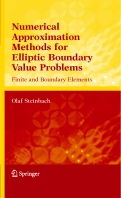ContentsOlaf Steinbach Numerical Approximation Methods for Elliptic Boundary Value Problems. Finite and Boundary Elements. Springer, New York, 390p., 2008. Springer's Book Homepage Although the aim of this book is to give a unified introduction into finite and boundary element methods, the main focus is on the numerical analysis of boundary integral and boundary element methods. Starting from the variational formulation of elliptic boundary value problems boundary integral operators and associated boundary integral equations are introduced and analyzed. By using finite and boundary elements corresponding numerical approximation schemes are considered. This textbook may serve as a basis for an introductory course in particular for boundary element methods including modern trends such as fast boundary element methods and efficient solution methods, as well as the coupling of finite and boundary element methods. Boundary Value Problems Function Spaces Variational Methods Variational Formulations of Boundary Value Problems Fundamental Solutions Boundary Integral Operators Boundary Integral Equations Approximation Methods Finite Elements Boundary Elements Finite Element Methods Boundary Element Methods Iterative Solution Methods Fast Boundary Element Methods Domain Decomposition Methods